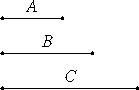# Proposition 22

If three numbers are in continued proportion, and the first is square, then the third is also square.

Let A, B, and C be three numbers in continued proportion, and let A the first be square.I say that C the third is also square.

VIII.20

Since between A and C there is one mean proportional number, B, therefore A and C are similar plane numbers. But A is square, therefore C is also square.

Therefore, if three numbers are in continued proportion, and the first is square, then the third is also square.

Q.E.D.

## Guide

This proposition is used in a few propositions in this and the next book starting with VIII.24.